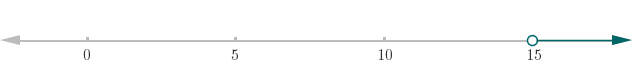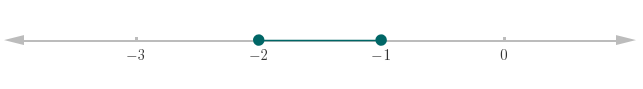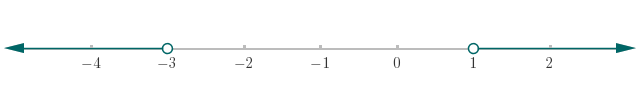Inequalities homework Help at TutorEye

## Inequalities Sample Questions

Question 1: Solve for unknown variable x, and write the answer using interval notation.Answer:Explanation:  Expand the bracket. And, rearrange the terms to solve forQuestion 2: Solve for q.Answer:Explanation:  Rearrange the terms to solve for q.

Question 3: Solve for r, and write the solution using interval notation.Answer:Explanation:  Expand the bracket. And, rewrite the terms to solve for.

Question 4: Solve for unknown variable x, and write the solution using interval notation.Answer:Explanation:  Subtract 2 from all parts of inequality.

Question 5: Solve for unknown variable x, and write the solution using interval notation.Answer:Explanation:  Add 3 to all parts of inequality.

Question 6: Solve for y.Answer:Explanation:  Add 3 to both sides of inequality.

Question 7: Solve for z, and write the answer using interval notation.Answer:Explanation:  Rearrange the terms to isolate the z.

Question 8: Solve for a. And, show the solution on the number line.Answer:Explanation:  Rearrange the terms to isolate the a.

Question 9: Solve for b. And, show the solution on the number line.Answer:Explanation:  Add 4 to all sides of the inequality.

Question 10: Solve for x, write the interval solution. And, show the solution on the number line.orAnswer:Explanation:  Solve each inequality.